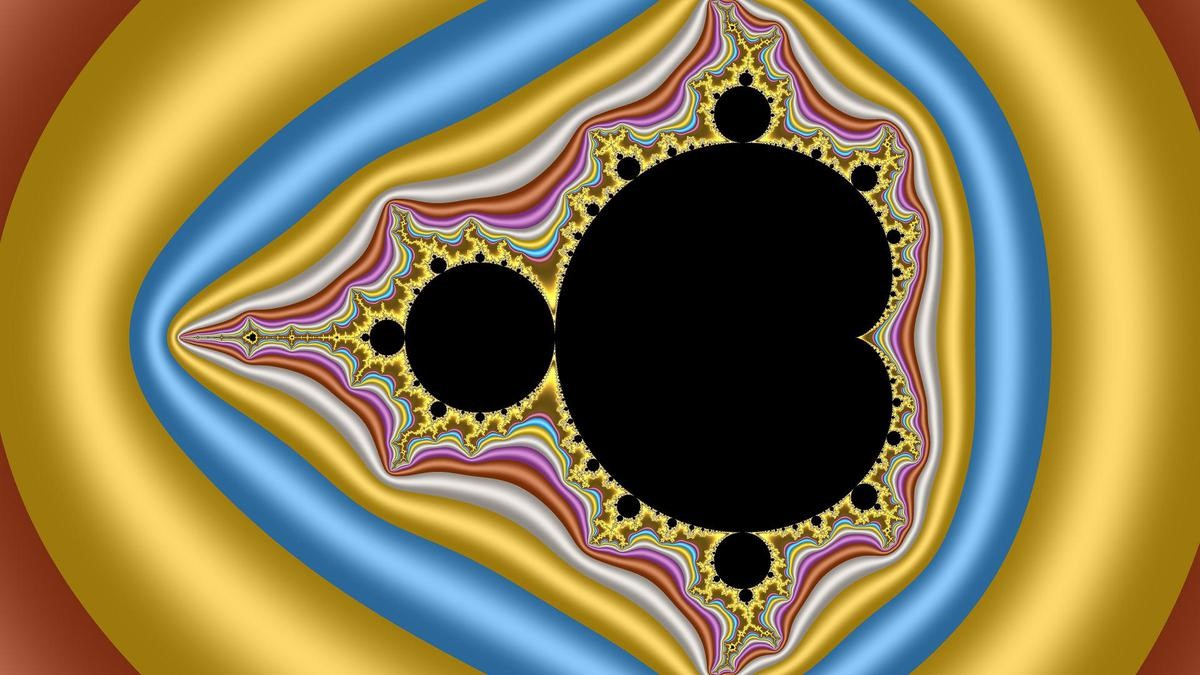# FRACTALS

21st November, 2023 Science and TechnologyContext

The utilization of fractal geometry in understanding the quantum realm introduces a unique perspective into the study of quantum systems in non-integer dimensions.

Details

Fractal Dimensions in Quantum Systems

• In quantum physics, Heisenberg's uncertainty principle limits precise knowledge of certain properties of particles.
• Physicists address this limitation using non-integer dimensions, known as fractal dimensions, to study quantum systems.
• This involves exploring dimensions like 1.55 or 1.58, allowing for a different viewpoint on quantum behavior.

Fractality and Quantum Behavior

• Fractals, known for self-similar patterns, offer a means to examine quantum systems in dimensions between one and two.
• For example, understanding how quantum systems might behave in non-integer or fractal dimensions leads to intriguing possibilities and insights into their behavior.Fractals in Nature and Quantum Realm

• Fractals are prevalent in nature, exhibiting self-similar patterns at various scales.
• In the quantum realm, studies have found fractal arrangements in materials like neodymium nickel oxide and graphene, showcasing fractal behavior in their internal structures and electron distributions.

Understanding Fractals

• Definition: Fractals are geometric shapes that display self-similar patterns at different scales. This means that when you zoom in or out on a fractal, you see similar or identical shapes repeating infinitely.
• Self-similarity: A defining characteristic of fractals is self-similarity, where a smaller portion of the fractal resembles the overall shape. This property allows for the infinite replication of patterns within the same structure.
• Mathematical Representation: Fractals can be represented by mathematical equations, iterative algorithms, or recursive processes. Common mathematical representations include the Mandelbrot set, Julia sets, Koch snowflake, Sierpinski triangle, and more.

Types of Fractals:

• Iterated Function Systems (IFS): These are sets of contracting transformations applied recursively to create fractals. Barnsley's fern and flame fractals are examples of IFS-generated fractals.
• Escape-Time Fractals: Generated by iterating a function on complex numbers and observing whether the resulting sequence remains within certain bounds. The Mandelbrot set and Julia sets are prominent examples of this type.
• L-systems: Used to model the growth processes of plants and trees. They generate fractal-like structures by iteratively applying production rules to create complex branching patterns.

Applications of Fractals:

• Art and Aesthetics: Fractals have inspired artists to create mesmerizing visual representations in paintings, sculptures, digital art, and music. Fractal art often showcases the intricate beauty of these mathematical forms.
• Computer Graphics: Fractals are extensively used in computer graphics for generating natural-looking terrains, textures, and realistic patterns. They play a vital role in creating visually stunning scenes in movies, video games, and simulations.
• Science and Nature: Fractal patterns are observed in natural phenomena such as coastlines, clouds, mountains, snowflakes, and biological systems. They help in modeling irregular structures found in nature.
• Data Compression and Signal Processing: Fractal compression techniques are used to efficiently compress and store images and signals by exploiting self-similarity properties.
• Fractals have diverse applications beyond physics, including data compression, antenna design, galaxy and planet pattern analysis, and biological systems' study, such as bacteria cultures.

Exploring Fractals:

• Visualization Tools: Various software tools and programs allow individuals to explore and create fractals. Fractint, Apophysis, Ultra Fractal, and Mandelbulb 3D are some popular tools for generating and visualizing fractals.
• Books and Resources: There are numerous books, online tutorials, and academic papers available for those interested in diving deeper into the mathematical concepts, algorithms, and applications of fractals.Conclusion

The integration of fractal geometry into the study of quantum systems provides a different lens through which physicists can analyze and interpret quantum phenomena in dimensions that deviate from classical integer values. This approach not only aids in understanding quantum behavior but also finds applications across diverse scientific domains, offering valuable insights into natural processes at both macroscopic and microscopic levels.

 PRACTICE QUESTION Q. Discuss the significance of fractals in modern science and their applications across different disciplines. How do fractals offer a unique perspective in understanding natural phenomena and technological advancements? Illustrate with relevant examples. (250 Words)